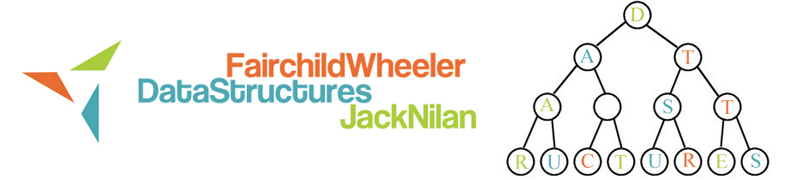# Objectives : Stacks and Queues

• understand concept of a queue as an ADT
• understand the concept of a stack as an ADT
• be able implement a stack using the stack interface
• understand the difference between an interface and a class
• understand concept of a queue as an ADT
• understand the concept of a stack as an ADT